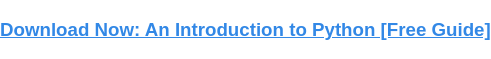# Python List Functions: 18 Definitions & ExamplesPublished:

Python is an excellent programming language for manipulating lists. It comes equipped with a series of built-in functions that you can use to alter and update lists of numbers, items, characters, and more.Below, we've curated a list of Python list functions that you can reference while coding your website. Use the list of functions as well as the interactive code module to program your site faster and become a more efficient website developer.## Python List Functions

This table will help you navigate to specific list functions. Copy the examples from the list of functions below into this interactive code module. Then click the play button (horizontal triangle) to see the output or the result of the function.

### 1. List Function: list()

The list function in Python creates an ordered list.

#### List Function Example:

sports = list(('baseball', 'football', 'soccer', 'hockey', 'basketball'))

print(sports)

Output:

Try with the code module

### 2. Next Function: next()

The next() function returns the item that appears next in an iterator.

#### Next Function Example:

mylist = iter(["blue", "red", "green"])

x = next(mylist)

print(x)

x = next(mylist)

print(x)

Output:

Blue

Red

Try with the code module

### 3. Range Function: range()

The range() function returns a sequence of numbers that starts at zero and ends at a specified number.

#### Range Function Example:

mylist = range(3)

for x in mylist:

print(x)

Output:

0

1

2

Try with the code module

mylist = {"dog", "cat", "bird"}

print(mylist)

Output:

{'cat', 'dog', 'bird', 'elephant'}

Try with the code module

### 5. Random Choice Function: choice()

The choice() function returns a random element from a sequence.

#### Random Choice Function Example:

import random

animals = ["bear", "squirrel", "bird", "deer", "hedgehog"]

print(random.choice(animals))

Output:

Deer

Try with the code module

### 6. Remove Function: remove()

The remove() function or method removes a specified element from a list.

#### Remove Function Example:

mylist = [1, 2, 3, 4, 5]

mylist.remove(4)

print(mylist)

Output:

[1, 2, 3, 5]

Try with the code module

### 7. Reverse Function: reverse()

The reverse() function or method reverses the order of elements in a list.

#### Reverse Function Example:

mylist = [1, 2, 3, 4, 5]

mylist.reverse()

print(mylist)

Output:

[5, 4, 3, 2, 1]

Try with the code module

### 8. Slice Function: slice()

The slice() functions returns a slice object which specifies where and how a sequence should be sliced.

#### Slice Function Example:

fruits = ("apple", "banana", "cherry", "kiwi", "date", "fig",)

x = slice(4)

print(fruits[x])

Output:

('apple', 'banana', 'cherry', 'kiwi')

Try with the code module

### 9. Sort Function: sort()

The sort() function or method organizes a list in ascending or descending order.

#### Sort Function Example:

mylist = [44, 3, 8, 16, 25]

mylist.sort()

print(mylist)

Output:

[3, 8, 16, 25, 44]

Try with the code module

### 10. Sorted Function: sorted()

The sorted() function returns a sorted list from an iterable object.

#### Sorted Function Example:

animals = ("dog", "cat", "bear", "deer", "koala", "rooster", "cow")

myvalue = sorted(animals)

print(myvalue)

Output:

['bear', 'cat', 'cow', 'deer', 'dog', 'koala', 'rooster']

Try with the code module

### 11. Filter Function: filter()

The filter function returns an iterator (element from an iterable) based on the output of a function testing the iterable or sequence.

#### Filter Function Example:

def greater_than_five(x):

if x > 5:

return True

nums = (1,4,6,2,9)

filter_nums = filter(greater_than_five, nums)

print(list(filter_nums))

Output:

6,9

// The example will print out the elements greater than 5 from the list, which would be 6 and 9.

Try with the code module

### 12. Pass a List to a Function: list()

The list() function is used to convert an iterable (such as a string, tuple or dictionary) into a list. It takes an iterable as an argument and returns a list with each element of the iterable as an item in the list.

#### Pass List to Function Example:

my_list = ["a", "b", "c"]

new_list = list(my_list)

print(new_list)

Output:

["a", "b", "c"]

Try with the code module

### 13. Append Function: append()

The append function adds a specified item to the end of a list.

#### Append Function Example:

fruits = ['Apple', 'Orange', 'Pear']

fruits.append('Banana')

Output:

['Apple', 'Orange', 'Pear', 'Banana']

Try with the code module

### 14. Clear Function: clear()

The clear() function removes all elements from a given list.

#### Clear Function Example:

your_list = [8, 4, 2, 1, 10, 15]

your_list.clear()

print(your_list)

Output:

[]

Try with the code module

### 15. Pop Function: pop()

The pop() function is used to remove an item from a list. It takes the index of the item as an argument and removes it from the list.

#### Pop Function Example:

my_list = ["a", "b", "c"]

my_list.pop(1)

print(my_list)

Output:

["a", "c"]

Try with the code module

### 16. Count Function: count()

The count() functions returns the number of times a given item appears in a list.

#### Count Function Example:

fruits = ['apple', 'banana', 'kiwi', 'apple', 'pear', 'strawberry', 'apple']

quantity = fruits.count('apple')

print(quantity)

Output:

3

Try with the code module

### 17. Extend Function: extend()

The extend() function adds all specified elements to the end of a list.

#### Extend Function Example:

my_list = [5, 6, 7, 8]

your_list = [1, 2, 3, 4]

your_list.extend(my_list)

print(your_list)

Output:

[1,2,3,4,5,6,7,8]

Try with the code module

### 18. Index Function: index()

The index() function searches for an element from the start of a list and returns the index of that element once found.

#### Index Function Example:

fruits = ['apple', 'pear', 'banana', 'kiwi']

index = fruits.index('pear')

print(index)

Output:

1

Try with the code moduleTopics: What Is Python?

## Related Articles

We're committed to your privacy. HubSpot uses the information you provide to us to contact you about our relevant content, products, and services. You may unsubscribe from these communications at any time. For more information, check out our Privacy Policy.

A guide for marketers, developers, and data analysts.